# Electron Orbit Magnetic Moment

From the classical expression for magnetic moment, m = IA, an expression for the magnetic moment from an electron in a circular orbit around a nucleus can be deduced. It is proportional to the angular momentum of the electron. The effective current is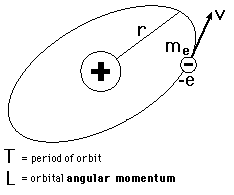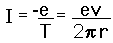which can be rewritten as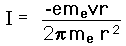so that the magnetic moment is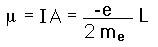### Show quantized form

Index

Schrodinger equation concepts

Hydrogen concepts

 HyperPhysics***** Quantum Physics R Nave
Go Back

# Orbital Magnetic Moment

The magnetic moment associated with an electron orbit is given byTaking into account the quantization of angular momentum for such orbits, the magnitude of the magnetic moment can be written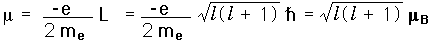A unit of magnetic moment called the "Bohr magneton" is introduced here.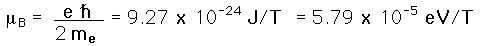The z-component of the magnetic moment is given by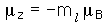Magnetic quantum number Zeeman effect Electron spin magnetic moment
Index

Schrodinger equation concepts

 HyperPhysics***** Quantum Physics R Nave
Go Back